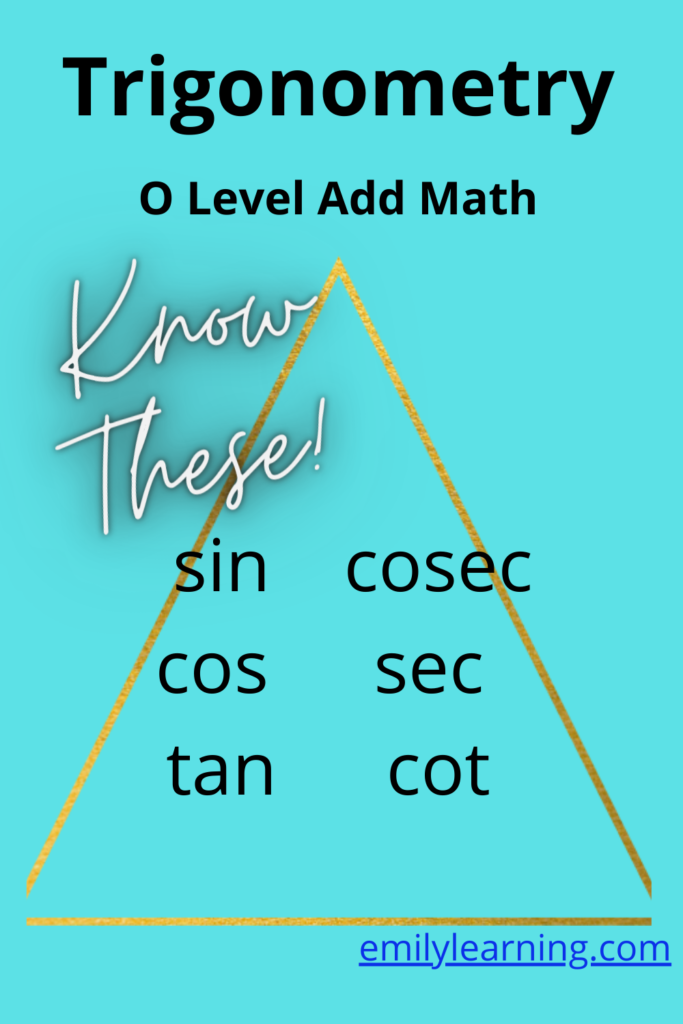# What you need to know for Trigonometry for O Level Additional MathematicsTrigonometry is a big topic in O Level Additional Mathematics. There are many different concepts in this topic Trigonometry.

For Additional Math, students need to also know the trigonometry concepts taught in elementary mathematics. These applying the ratio of sine, cosine, and tangent to right-angle triangles and the use of Pythagora’s theorem. In addition, students are expected to know the sine rule and cosine rule. Don’t worry about memorizing the sine and cosine rules since they are available in the formula booklet.

You will also see trigonometry in the chapters on integration and differentiation. I’ll talk more about trigonometry in integration and differentiation in other posts.

For O Level Additional Mathematics, students are expected to know the following for Trigonometry:

• Use of sin, cos, tan, cosec, sec, and cot
• principal values of sin-1, cos-1 and tan-1
• Be familiar with angles in degrees and radians
• Exact values of the trigonometric functions for special angles (30o, 45o and 60o)
• Graphs of sine, cosine and tangent, and the period and amplitude of such graphs
• Use of trigonometric identities for proving
•  Simplify trigonometric expressions using trigonometric identities
• Solving trigonometric equations
• R- formula

## Want to learn O Level Additional Math on-demand?

Check out our on-demand O Level Additional Math course here.